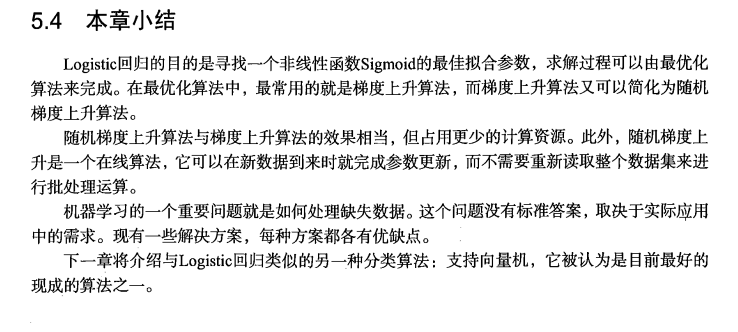# Sail

### 概述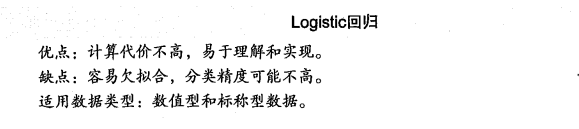$$图(1)$$

$f(x) = w_1 x_1 + w_2 x_2 + … + w_n x_n + b$

$f(x) = w^T + b$

$h\theta(x) = g(\theta^T * x)$
$g(z) = \frac{1}{1+e^-z}$

$z = w_0 x_0 + w_1 x_1 + w_2 x_2 + … + w_n x_n$

$h\theta(x) = g(\theta_0 + \theta_1 x_1 + \theta_2 x_2 + …)$

### 梯度上升(下降)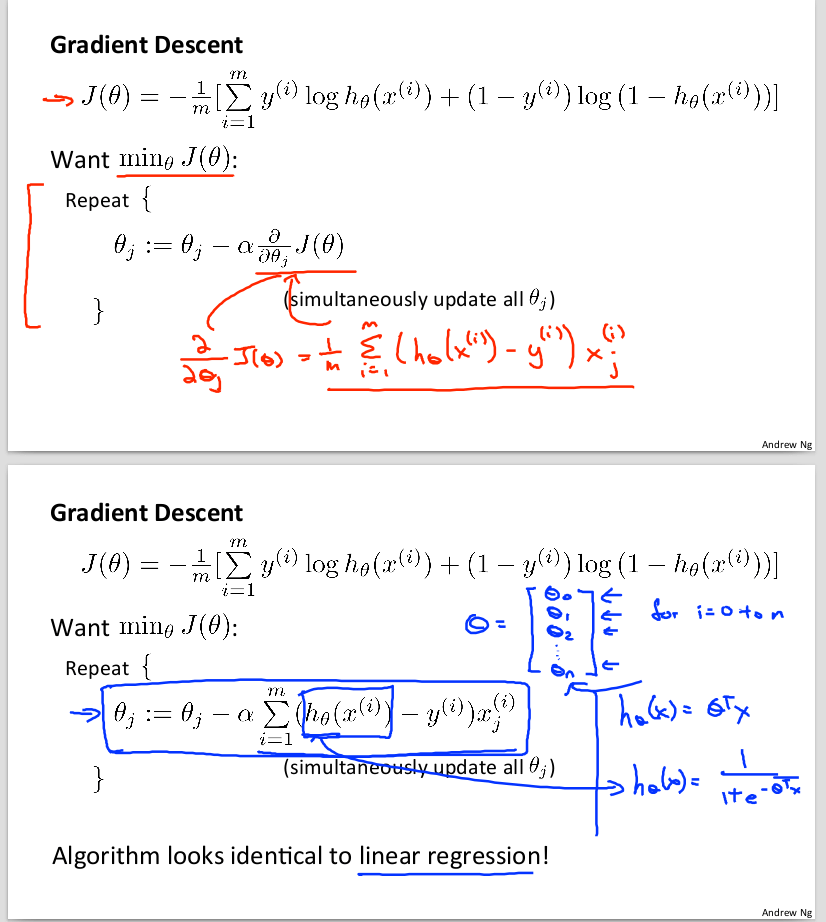$$图(2)$$

计算整个数据集的梯度

### 分析数据: 画出决策边界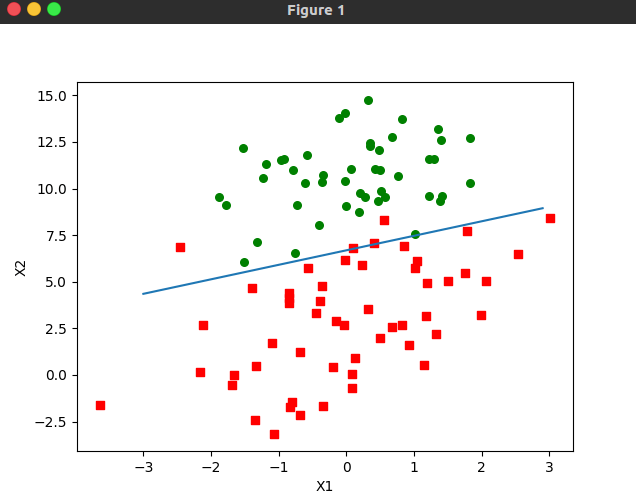$$图(3) 梯度上升算法在500次迭代后得到的Logistic回归最佳拟合直线$$

### 训练算法: 随机梯度上升

计算该样本的梯度

1. 后者的变量h和误差error都是向量,而前者则全是数值
2. 前者没有矩阵转置过程,所有变量的数据类型都是Numpy数组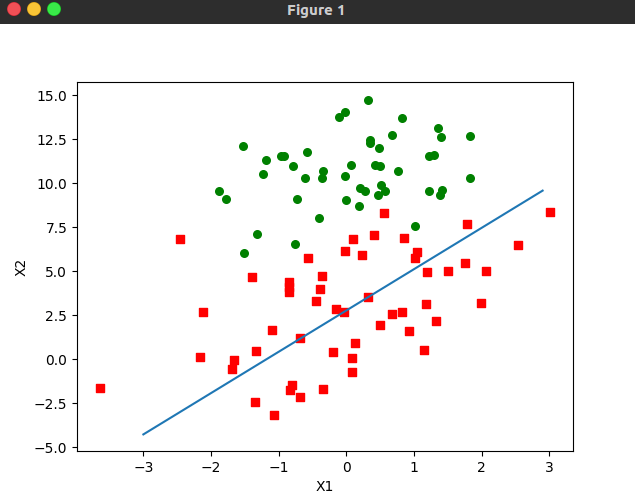$$图(4)随机梯度上升算法在上述数据集上的执行结果,最佳拟合执行并非最佳分类线$$$$图(5)$$

### 改进的随机梯度上升算法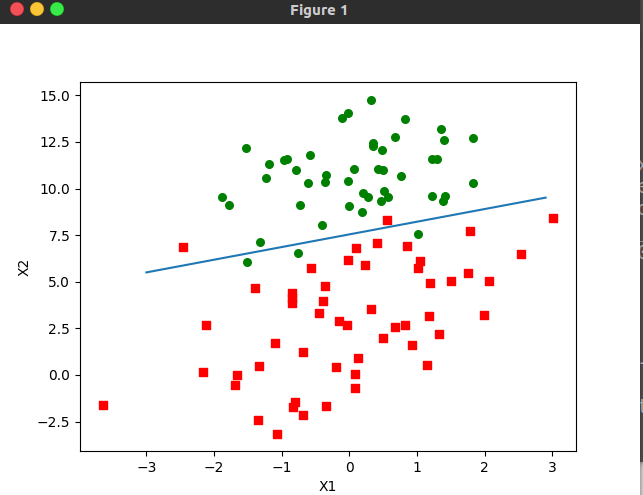### 示例: 从疝气病预测病马的死亡率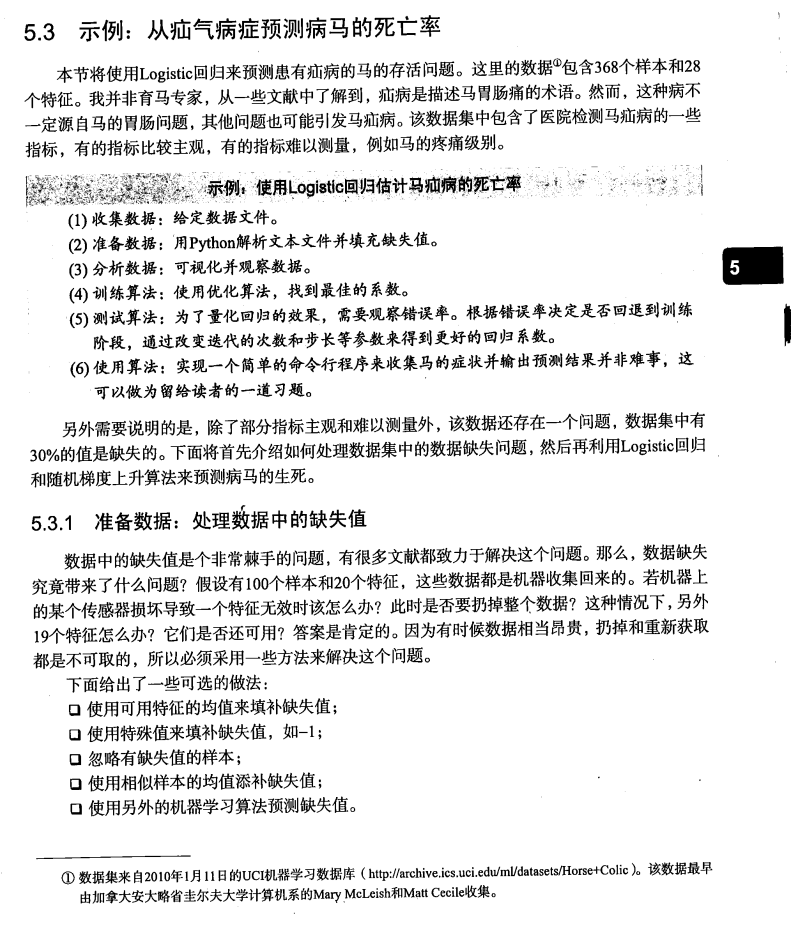### 总结